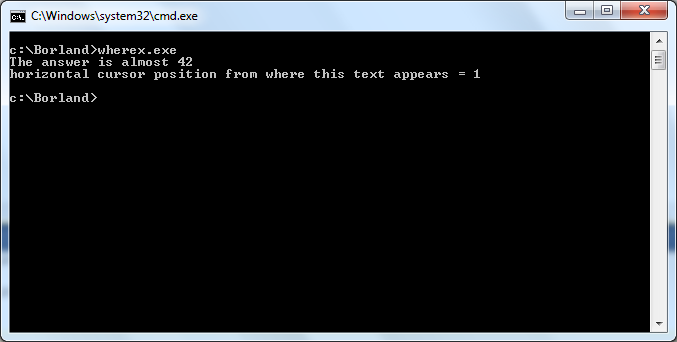### Programming Reference/Librarys

Q&A is closed

c:conio.h:wherex# wherex

`int wherex();`

### description of wherex

return current horizontal cursor position

```#include<stdio.h>
#include<conio.h>

int main( void )
{
int x;
x = wherex();
printf("horizontal cursor position from where this text appears = %d\n",x);
return 0;
}```

## output of wherex example

```  The answer is almost 42
horizontal cursor position from where text appears = 1```## on the occasion of the current invasion of Russia in Ukraine